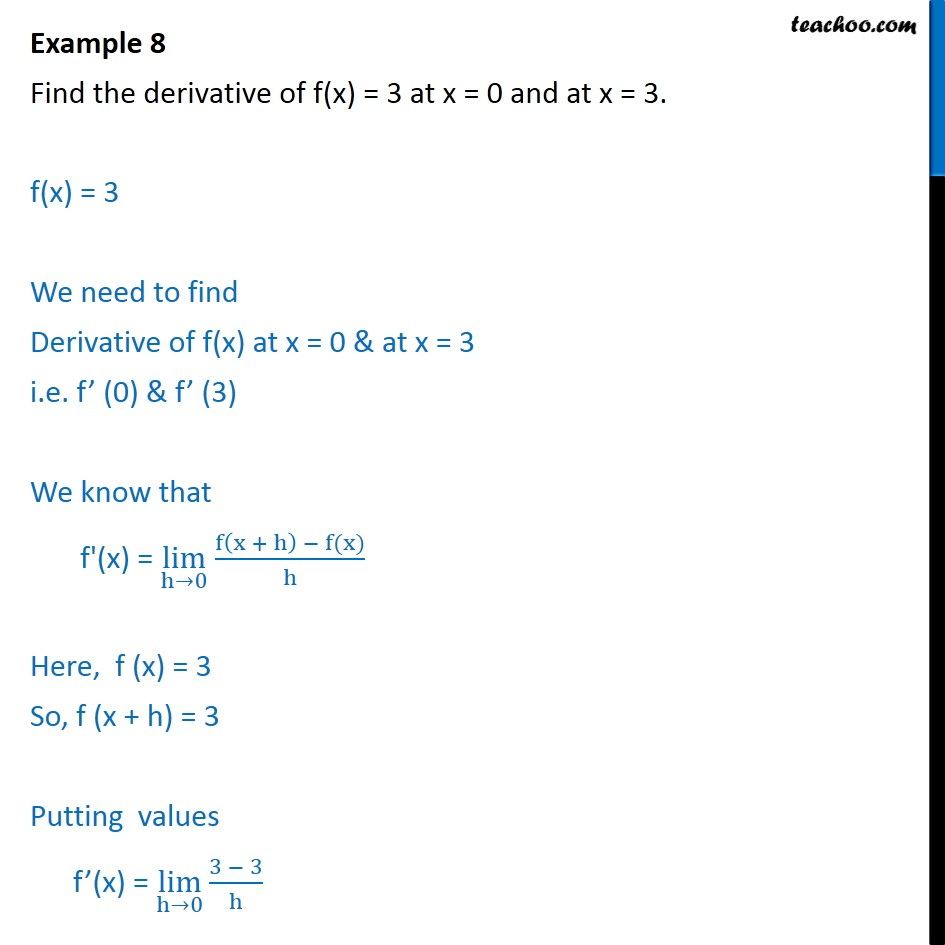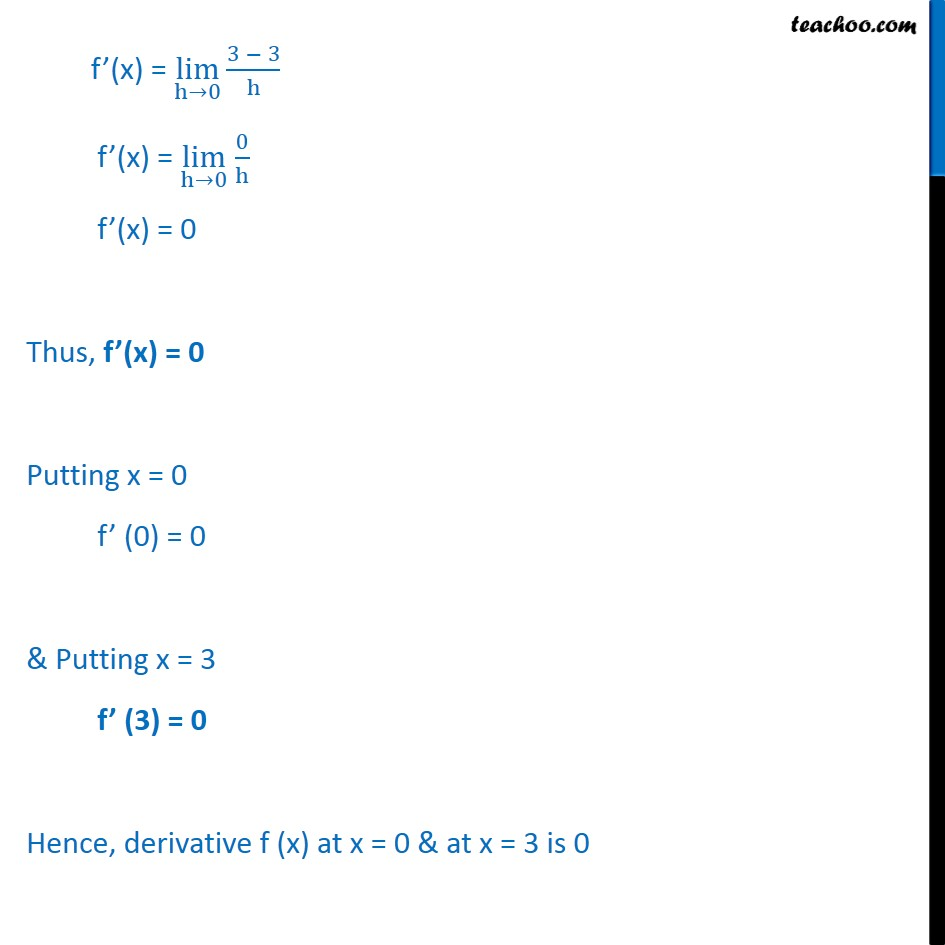1. Chapter 13 Class 11 Limits and Derivatives
2. Concept wise
3. Derivatives by 1st principle - At a point

Transcript

Example 8 Find the derivative of f(x) = 3 at x = 0 and at x = 3. f(x) = 3 We need to find Derivative of f(x) at x = 0 & at x = 3 i.e. f (0) & f (3) We know that f'(x) = lim h 0 f x + h f(x) h Here, f (x) = 3 So, f (x + h) = 3 Putting values f (x) = lim h 0 3 3 h f (x) = lim h 0 3 3 h f (x) = lim h 0 0 h f (x) = 0 Thus, f (x) = 0 Putting x = 0 f (0) = 0 & Putting x = 3 f (3) = 0 Hence, derivative f (x) at x = 0 & at x = 3 is 0

Derivatives by 1st principle - At a point

About the AuthorDavneet Singh
Davneet Singh is a graduate from Indian Institute of Technology, Kanpur. He has been teaching from the past 10 years. He provides courses for Maths and Science at Teachoo.